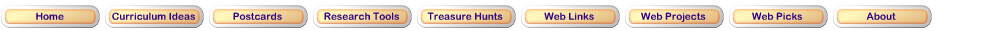Step 1: Using pattern blocksHave you ever used pattern blocks to think about fractions? This exploration using the Math Playground applet will show you how!Step 2: Multiplying fractionsNow that you are familiar with pattern blocks, you can use them to multiply fractions and whole numbers. As you work on this exploration, think about what you already know about how whole number multiplication works. How is multiplying fractions different than multiplying whole numbers? How is it the same?Step 3: Dividing fractionsIn this final exploration, you will use the Math Playground pattern blocks applet to learn how to divide fractions. How is dividing fractions different than dividing whole numbers? How is it the same? What strategiesStep 4: Worksheets 1 and 2Here are your questions for this lesson. Worksheet 1 and Worksheet 2 Your teacher may ask you to work on this assignment in class, or it may be a part of your homework.Updated May 25, 2017
© 1996 - 2009 Linda C. Joseph